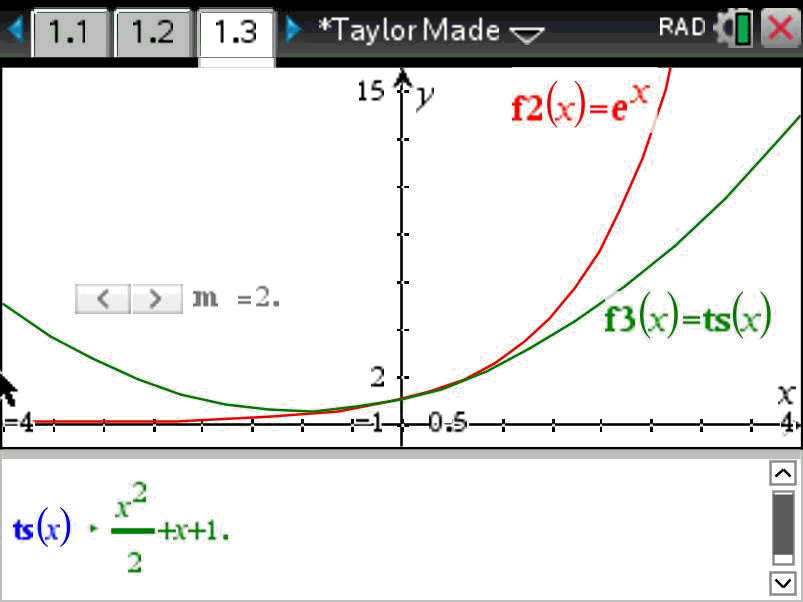# Activities

•• ##### AuthorAust Senior

45 Minutes

• ##### Device
•TI-Nspire™ CX CAS
• ##### Software

TI-Nspire™ CAS

4.5

• ##### Report an Issue

Taylor Polynomials#### Activity Overview

The modulus and argument form of a complex number can be written in two ways, but how are they connected? ‘CIS’ format is a straight forward application of trigonometry, but what about e ? In this activity students explore the Taylor expansion of ex and compare it with the Taylor expansion of cos(x) + sin(x) revealing a small difference relating to an alternating sequence of negative signs which can be adjusted using i. The activity removes the mystery and replaces it with a beautifully connected piece of mathematics

#### Objectives

• Complex numbers in polar form (modulus and argument)
• Proof of basic identities involving modulus and argument
• Proof of DeMoivre’s Theorem for integral powers

#### Vocabulary

• Taylor Series,
• Taylor Polynomial,
• Complex numbers in rectangular form,
• Complex numbers in polar form
• Modulus
• Argument

#### About the Lesson

A Taylor polynomial is a finite number of terms from a Taylor series.  In this activity Taylor polynomials are used that approximate exponential and trigonometric functions.  The introduction of the complex number i shows how the trigonometric functions can be added to produce the exponential function and therefore making the link between the two types of polar representation of a complex number.